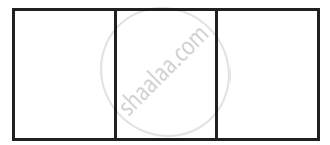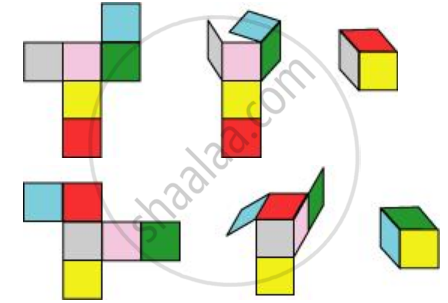Share

# Here is an Incomplete Net for Making a Cube. Complete It in at Least Two Different Ways. Remember that a Cube Has Six Faces. How Many Are There in the Net Here? (Give Two Separate Diagrams. If You Like, You May Use a Squared Sheet for Easy Manipulation.) - CBSE Class 7 - Mathematics

ConceptConcept of Faces, Edges and Vertices

#### Question

Here is an incomplete net for making a cube. Complete it in at least two different ways. Remember that a cube has six faces. How many are there in the net here? (Give two separate diagrams. If you like, you may use a squared sheet for easy manipulation.)#### Solution

There are 3 faces in the given net. The given net can be completed as follows.Is there an error in this question or solution?

#### APPEARS IN

NCERT Solution for Mathematics for Class 7 (2018 to Current)
Chapter 15: Visualising Solid Shapes
Ex. 15.10 | Q: 4 | Page no. 282
Solution Here is an Incomplete Net for Making a Cube. Complete It in at Least Two Different Ways. Remember that a Cube Has Six Faces. How Many Are There in the Net Here? (Give Two Separate Diagrams. If You Like, You May Use a Squared Sheet for Easy Manipulation.) Concept: Concept of Faces, Edges and Vertices.
S